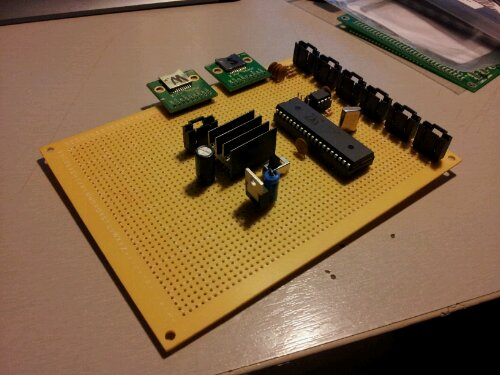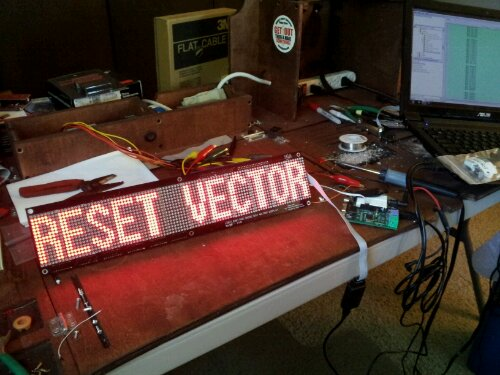# Code tweaking on Reset_Vector

So I was still getting glitches on my inputs for Reset_Vector. I couldn’t figure out what was causing it because it would happen randomly and I couldn’t repeat the problem. The false inputs caused issues with ball triggering, score detection, and playfield actions. I figured it had to be glitches on the lines caused by EMF or something on the input driver. I knew it was the input driver cause when a solenoid fired randomly like the pop bumper it would register a hit which means that the microcontroller detected a hit.

To fix this issue I added a simple debouncer routine. Basically it makes sure a input is pressed for at least 2 Kernel cycles before saying that it was a hit. This keeps random spikes from registering as hits.

What it does is read in the inputs into a variable called new_inputs. The new_inputs then gets anded with a variable called old_inputs and the result is the inputs that where held for two kernel cycles. Then the new_inputs is copied into old_inputs for the next cycle to happen.

This system can be easily expanded to 3,4,ect cycles so the debouncer is extremely configurable in its sensitivity.

# 16×96 DMD Code for Reset Vector

While developing the larger 36×128 DMD I ran out of registers and logic elements in the FPGA I was using. I could have switched to a larger FPGA but that would put the final product out of the price range I was aiming for. FPGAs have loads of dedicated ram so I decided to move the display memory from registers to a chunk of block ram. To test to see if the RAM code works I wrote a program for the 16×96 display in RESET_VECTOR. It took a bit of tweaking as I have never used block ram before but I was able to get it working.

``````
module LED_Matrix_16x96
(
clk,row,col,SDRAM_CS,SRAM_CS,LAT,INPUT,SCLK
);``````

``````input  wire    clk;
input  wire    LAT;
input  wire    INPUT;
input  wire    SCLK;

output  reg [0:15]  row;
output  reg [95:0]  col;
output  reg [0:0]   SDRAM_CS;
output  reg [0:0]   SRAM_CS;

reg [24:0]  clk_slow;
reg [4:0]  row_cnt;
reg [1535:0]  col_buffer;

reg  [95:0]  disp_mem_data;
reg      disp_mem_wren;
wire  [95:0]  disp_mem_q;

reg [3:0]  sel;

``````
``````initial
begin
row &lt;= 16'b0000000000000001;
col &lt;= 16'b0000000000000000;
SDRAM_CS &lt;= 1'b0;
SRAM_CS &lt;= 1'b0;
clk_slow &lt;= 16'b0000000000000000;
row_cnt &lt;= 5'b00000;
disp_mem_wren &lt;= 0;
sel &lt;= 4'b0000;
end

always @(posedge clk)
begin
clk_slow &lt;= clk_slow + 1'b1;

if(!LAT &amp; row_cnt == 5'b01111)
begin
case(sel)
4'b0000:
begin
disp_mem_wren &lt;= 1'b1;
disp_mem_data &lt;= col_buffer[1535:1440];
sel &lt;= 4'b0001;
end
4'b0001:
begin
disp_mem_wren &lt;= 1'b1;
disp_mem_data &lt;= col_buffer[1439:1344];
sel &lt;= 4'b0010;
end
4'b0010:
begin
disp_mem_wren &lt;= 1'b1;
disp_mem_data &lt;= col_buffer[1343:1248];
sel &lt;= 4'b0011;
end
4'b0011:
begin
disp_mem_wren &lt;= 1'b1;
disp_mem_data &lt;= col_buffer[1247:1152];
sel &lt;= 4'b0100;
end
4'b0100:
begin
disp_mem_wren &lt;= 1'b1;
disp_mem_data &lt;= col_buffer[1151:1056];
sel &lt;= 4'b0101;
end
4'b0101:
begin
disp_mem_wren &lt;= 1'b1;
disp_mem_data &lt;= col_buffer[1055:960];
sel &lt;= 4'b0110;
end
4'b0110:
begin
disp_mem_wren &lt;= 1'b1;
disp_mem_data &lt;= col_buffer[959:864];
sel &lt;= 4'b0111;
end
4'b0111:
begin
disp_mem_wren &lt;= 1'b1;
disp_mem_data &lt;= col_buffer[863:768];
sel &lt;= 4'b1000;
end
4'b1000:
begin
disp_mem_wren &lt;= 1'b1;
disp_mem_data &lt;= col_buffer[767:672];
sel &lt;= 4'b1001;
end
4'b1001:
begin
disp_mem_wren &lt;= 1'b1;
disp_mem_data &lt;= col_buffer[671:576];
sel &lt;= 4'b1010;
end
4'b1010:
begin
disp_mem_wren &lt;= 1'b1;
disp_mem_data &lt;= col_buffer[575:480];
sel &lt;= 4'b1011;
end
4'b1011:
begin
disp_mem_wren &lt;= 1'b1;
disp_mem_data &lt;= col_buffer[479:384];
sel &lt;= 4'b1100;
end
4'b1100:
begin
disp_mem_wren &lt;= 1'b1;
disp_mem_data &lt;= col_buffer[383:288];
sel &lt;= 4'b1101;
end
4'b1101:
begin
disp_mem_wren &lt;= 1'b1;
disp_mem_data &lt;= col_buffer[287:192];
sel &lt;= 4'b1110;
end
4'b1110:
begin
disp_mem_wren &lt;= 1'b1;
disp_mem_data &lt;= col_buffer[191:96];
sel &lt;= 4'b1111;
end
4'b1111:
begin
disp_mem_wren &lt;= 1'b1;
disp_mem_data &lt;= col_buffer[95:0];
sel &lt;= 4'b0000;
end
default:
begin
sel &lt;= 4'b0000;
disp_mem_wren &lt;= 1'b0;
end
endcase
end
else
begin
disp_mem_wren &lt;= 1'b0;
sel &lt;= 4'b0000;
end
end

always @(posedge clk_slow)
begin
if(row_cnt &gt; 5'b01111)
begin
row_cnt &lt;= 5'b00000;
end
col &lt;= disp_mem_q;
row_cnt &lt;= row_cnt + 1'b1;
row &lt;= {row,row[0:14]};

end

always @(posedge SCLK)
begin
if(LAT)
begin
col_buffer &lt;= {col_buffer[1534:0],INPUT};
end
end

display_ram disp_mem_inst (
.clock  (clk),
.data   (disp_mem_data),
.wren   (disp_mem_wren),
.q      (disp_mem_q)
);

endmodule
``````

# More Verilog Code

Sorry for the massive amount of code recently. To test to see if the RAM code works I wrote a program for the 16×96 display in RESET_VECTOR. If this works then I will order the PCB for the 32×128 DMD. If anyone has any suggestions please feel free to comment. This is the first time I have tried interfacing RAM on an FPGA before.

``````
module LED_Matrix_16x96
(
clk,row,col,SDRAM_CS,SRAM_CS,LAT,INPUT,SCLK
);

input  wire    clk;
input  wire    LAT;
input  wire    INPUT;
input  wire    SCLK;

output  reg [0:15]  row;
output  reg [95:0]  col;
output  reg [0:0]   SDRAM_CS;
output  reg [0:0]   SRAM_CS;

reg [24:0]  clk_slow;
reg [4:0]  row_cnt;
reg [1535:0]  col_buffer;

reg  [95:0]  disp_mem_data;
reg      disp_mem_wren;
wire  [95:0]  disp_mem_q;

reg [3:0]  sel;

initial
begin
row <= 16'b0000000000000001;
col <= 16'b0000000000000000;
SDRAM_CS <= 1'b0;
SRAM_CS <= 1'b0;
clk_slow <= 16'b0000000000000000;
row_cnt <= 5'b00000;
disp_mem_wren <= 0;
sel <= 4'b0000;
end

always @(posedge clk)
begin
clk_slow <= clk_slow + 1'b1;

if(!LAT & row_cnt == 5'b01111)
begin
case(sel)
4'b0000:
begin
disp_mem_wren <= 1'b1;
disp_mem_data <= col_buffer[1535:1440];
sel <= 4'b0001;
end
4'b0001:
begin
disp_mem_wren <= 1'b1;
disp_mem_data <= col_buffer[1439:1344];
sel <= 4'b0010;
end
4'b0010:
begin
disp_mem_wren <= 1'b1;
disp_mem_data <= col_buffer[1343:1248];
sel <= 4'b0011;
end
4'b0011:
begin
disp_mem_wren <= 1'b1;
disp_mem_data <= col_buffer[1247:1152];
sel <= 4'b0100;
end
4'b0100:
begin
disp_mem_wren <= 1'b1;
disp_mem_data <= col_buffer[1151:1056];
sel <= 4'b0101;
end
4'b0101:
begin
disp_mem_wren <= 1'b1;
disp_mem_data <= col_buffer[1055:960];
sel <= 4'b0110;
end
4'b0110:
begin
disp_mem_wren <= 1'b1;
disp_mem_data <= col_buffer[959:864];
sel <= 4'b0111;
end
4'b0111:
begin
disp_mem_wren <= 1'b1;
disp_mem_data <= col_buffer[863:768];
sel <= 4'b1000;
end
4'b1000:
begin
disp_mem_wren <= 1'b1;
disp_mem_data <= col_buffer[767:672];
sel <= 4'b1001;
end
4'b1001:
begin
disp_mem_wren <= 1'b1;
disp_mem_data <= col_buffer[671:576];
sel <= 4'b1010;
end
4'b1010:
begin
disp_mem_wren <= 1'b1;
disp_mem_data <= col_buffer[575:480];
sel <= 4'b1011;
end
4'b1011:
begin
disp_mem_wren <= 1'b1;
disp_mem_data <= col_buffer[479:384];
sel <= 4'b1100;
end
4'b1100:
begin
disp_mem_wren <= 1'b1;
disp_mem_data <= col_buffer[383:288];
sel <= 4'b1101;
end
4'b1101:
begin
disp_mem_wren <= 1'b1;
disp_mem_data <= col_buffer[287:192];
sel <= 4'b1110;
end
4'b1110:
begin
disp_mem_wren <= 1'b1;
disp_mem_data <= col_buffer[191:96];
sel <= 4'b1111;
end
4'b1111:
begin
disp_mem_wren <= 1'b1;
disp_mem_data <= col_buffer[95:0];
sel <= 4'b0000;
end
default:
begin
sel <= 4'b0000;
disp_mem_wren <= 1'b0;
end
endcase
end
else
begin
disp_mem_wren <= 1'b0;
sel <= 4'b0000;
end
end

always @(posedge clk_slow)
begin
if(row_cnt > 5'b01111)
begin
row_cnt <= 5'b00000;
end
col <= disp_mem_q;
row_cnt <= row_cnt + 1'b1;
row <= {row,row[0:14]};

end

always @(posedge SCLK)
begin
if(LAT)
begin
col_buffer <= {col_buffer[1534:0],INPUT};
end
end

display_ram disp_mem_inst (
.clock  (clk),
.data   (disp_mem_data),
.wren   (disp_mem_wren),
.q      (disp_mem_q)
);

endmodule
``````

# Reset Vector: New main boardA work in progress. Some of the new features include a socketable Propeller Chip, lockable headers, and separate power lines for the microcontroller and other sub systems. These changes will make the system easier to fix and prevent future problems.

# RESET VECTOR: DMD 16×96 Single Line Test

I finally finished building the Dot Matrix Display for Reset Vector. The Matrixing is run by a FPGA. The data is shifted in by the main propeller microcontroller. Currently the only code on the propeller is the single line text generator. I have a double line text generator and a animation driver that will load bitmaps off an SD card.

The Code will be up tomorrow.

# Reset Vector : LED DMD done!Just got it working.

# LDMD 16×96 PCB and FPGA working!

I soldered on what parts I had on hand and got the DMD display working today. Will upload the code after I get all the modules soldered on.# LDMD 16×16 Propeller Comm Test

Just finished the DMD (Dot Matrix Display) test for RESET_VECTOR. Since the Propeller is to slow to do the matrixing I am going to use a FPGA to do it. The propeller will send the data over a serial connection into a frame buffer on the FPGA. When all the data is on the FPGA the FPGA will update its matrixing buffer.

I have the Propeller to FPGA communication protocol written and tested as well. The Propeller loads the Data off the SD card and sends it serially to the FPGA.